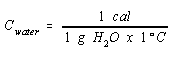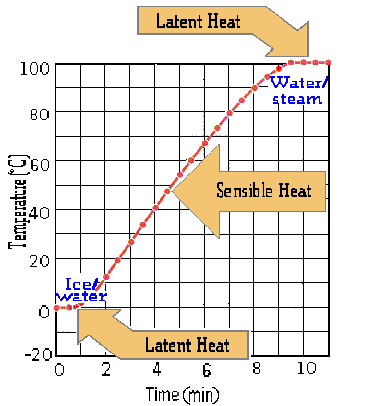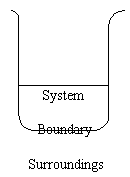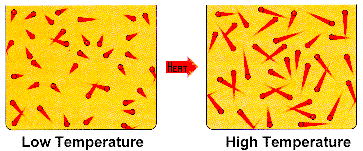Heat

Heat

 Heat Heat Capacity Specific Heat Latent Heat Kinetic Molecular Theory

Heat

Heat is a way of transferring energy between a system and its surroundings that often, but not always, changes the temperature of the system. Heat is not conserved, it can be either created or destroyed. In the metric system, heat is measured in units of calories, which are defined as the amount of heat required to raise the temperature of one gram of water from 14.5oC to 15.5oC.

In the SI system, the unit of heat is the joule.Heat Capacity

The heat capacity of a substance is the amount of heat required to raise the temperature of a defined amount of pure substances by one degree (Celsius or Kelvin). The calorie was defined so that the heat capacity of water was equal to one.Specific Heat

The specific heat of a substance is the number of calories needed to raise the temperature of one gram by 1oC. Because one degree on the Celsius scale is equal to one Kelvin, specific heats in the metric system can be reported in units of either cal/g-oC or cal/g-K. The units of specific heat in the SI system are J/g-K. Because there are 4.184 joules in a calorie, the specific heat of water is 4.184 J/g-K.

The ease with which a substance gains or loses heat can also be described in terms of its molar heat capacity, which is the heat required to raise the temperature of one mole of the substance by either 1oC or 1 K. In the metric system, the units of molar heat capacities are therefore either cal/mol-oC or cal/mol-K. In the SI system the units of molar heat capacities are J/mol-K.

 Practice Problem 1:Use the following equality to calculate the specific heat of water in J/g-K and the molar heat capacity of water in J/mol-K. 1 cal = 4.184 J Click here to check your answer to Practice Problem 1 Click here to see a solution to Practice Problem 1Latent Heat

When ice is heated, the heat that initially enters the system is used to melt the ice. As the ice melts the temperature remains constant at 0oC. The amount of heat required to melt the icehas historically been called the latent heat of fusion. Once the ice has melted, the temperature of the water slowly increases from 0oC to 100oC. But once the water starts to boil, the heat that enters the sample is used to convert the liquid into a gas and the temperature of the sample remains constant until the liquid evaporates. The amount of heat required to boil, or vaporize, the liquid has historically been called the latent heat of vaporization.More than 200 years ago, Joseph Black distinguished between sensible heat and latent heat. Heat that raises the temperature of the system can be sensed, but heat that results in a change in the state of the system from solid to liquid or from liquid to gas is latent. Like the latent image on photograph film that hasn't been developed or latent fingerprints that can't be seen with the naked eye, latent heat is heat that enters the system without changing the temperature of the system.Heat and The Kinetic Molecular Theory

The system is the small portion of the universe in which we are interested, such as the water in a beaker or a gas trapped in a piston and cylinder, as shown in the figures below. The surroundings are everything elsein other words, the rest of the universe.The system and its surroundings are separated by a boundary. Heat is transferred across the boundary between a system and its surroundings.

One of the fundamental principles of the kinetic theory is the assumption that the average kinetic energy of a collection of gas particles depends on the temperature of the gas and nothing else. A gas becomes warmer if and only if the average kinetic energy of the gas particles increases. Heat, when it raises the temperature of a system, produces an increase in the speed with which the particles of the system move, as shown in the figure below.